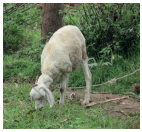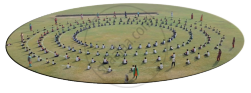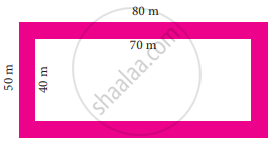Tamil Nadu Board of Secondary EducationSSLC (English Medium) Class 7th

# Tamil Nadu Board Samacheer Kalvi solutions for Class 7th Mathematics Term 2 Answers Guide chapter 2 - Measurements [Latest edition]

#### Chapters## Chapter 2: Measurements

Exercise 2.1Exercise 2.2Exercise 2.3Exercise 2.4
Exercise 2.1 [Pages 27 - 28]

### Tamil Nadu Board Samacheer Kalvi solutions for Class 7th Mathematics Term 2 Answers Guide Chapter 2 MeasurementsExercise 2.1 [Pages 27 - 28]

Exercise 2.1 | Q 1. (i) | Page 27

Find the missing values in the following table for the circles with radius (r), diameter (d) and Circumference (C).

 radius (r) diameter (d) Circumference (C) 15 cm
Exercise 2.1 | Q 1. (ii) | Page 27

Find the missing values in the following table for the circles with radius (r), diameter (d) and Circumference (C).

 radius (r) diameter (d) Circumference (C) 1760 cm
Exercise 2.1 | Q 1. (iii) | Page 27

Find the missing values in the following table for the circles with radius (r), diameter (d) and Circumference (C).

 radius (r) diameter (d) Circumference (C) 24 m
Exercise 2.1 | Q 2. (i) | Page 27

Diameters of different circles are given below. Find their circumference (Take π = 22/7)

d = 70 cm

Exercise 2.1 | Q 2. (ii) | Page 27

Diameters of different circles are given below. Find their circumference (Take π = 22/7)

d = 56 m

Exercise 2.1 | Q 2. (iii) | Page 27

Diameters of different circles are given below. Find their circumference (Take π = 22/7)

d = 28 mm

Exercise 2.1 | Q 3. (i) | Page 27

Find the circumference of the circles whose radii are given below.

49 cm

Exercise 2.1 | Q 3. (ii) | Page 27

Find the circumference of the circles whose radii are given below.

91 mm

Exercise 2.1 | Q 4 | Page 27

The diameter of a circular well is 4.2 m. What is its circumference?

Exercise 2.1 | Q 5 | Page 28

The diameter of the bullock cart wheel is 1.4 m. Find the distance covered by it in 150 rotations?

Exercise 2.1 | Q 6 | Page 28

A ground is in the form of a circle whose diameter is 350 m. An athlete makes 4 revolutions. Find the distance covered by the athlete

Exercise 2.1 | Q 7 | Page 28

A wire of length 1320 cm is made into circular frames of radius 7 cm each. How many frames can be made?

Exercise 2.1 | Q 8 | Page 28

A Rose garden is in the form of circle of radius 63 m. The gardener wants to fence it at the rate of ₹ 150 per metre. Find the cost of fencing?

#### Objective type questions

Exercise 2.1 | Q 9 | Page 28

Formula used to find the circumference of a circle is

• 2πr units

• πr2 + 2r units

• πr2 sq.units

• πr3 cu.units

Exercise 2.1 | Q 10 | Page 28

In the formula, C = 2πr, ‘r’ refers to

• circumference

• area

• rotation

Exercise 2.1 | Q 11 | Page 28

If the circumference of a circle is 82π, then the value of ‘r’ is

• 41 cm

• 82 cm

• 21 cm

• 20 cm

Exercise 2.1 | Q 12 | Page 28

Circumference of a circle is always

• three times of its diameter

• more than three times of its diameter

• less than three times of its diameter

• three times of its radius

Exercise 2.2 [Pages 33 - 34]

### Tamil Nadu Board Samacheer Kalvi solutions for Class 7th Mathematics Term 2 Answers Guide Chapter 2 MeasurementsExercise 2.2 [Pages 33 - 34]

Exercise 2.2 | Q 1 | Page 33

Find the area of the dining table whose diameter is 105 cm

Exercise 2.2 | Q 2 | Page 33

Calculate the area of the shotput circle whose radius is 2.135 m.

Exercise 2.2 | Q 3 | Page 33

A sprinkler placed at the centre of a flower garden sprays water covering a circular area. If the area watered is 1386 cm2, find its radius and diameter

Exercise 2.2 | Q 4 | Page 33

The circumference of a circular park is 352 m. Find the area of the park

Exercise 2.2 | Q 5 | Page 33

In a grassland, a sheep is tethered by a rope of length 4.9 m. Find the maximum area that the sheep can grazeExercise 2.2 | Q 6 | Page 33

Find the length of the rope by which a bull must be tethered in order that it may be able to graze an area of 2464 m2

Exercise 2.2 | Q 7 | Page 33

Lalitha wants to buy a round carpet of radius is 63 cm for her hall. Find the area that will be covered by the carpet

Exercise 2.2 | Q 8 | Page 33

Thenmozhi wants to level her circular flower garden whose diameter is 49 m at the rate of ₹ 150 per m2 Find the cost of levelling

Exercise 2.2 | Q 9 | Page 34

The floor of the circular swimming pool whose radius is 7 m has to be cemented at the rate of ₹ 18 per m2. Find the total cost of cementing the floor

#### Objective type questions

Exercise 2.2 | Q 10 | Page 34

The formula used to find the area of the circle is ________ sq.units

• 4πr2

• πr2

• 2πr2

• πr2 + 2r

Exercise 2.2 | Q 11 | Page 34

The ratio of the area of a circle to the area of its semicircle is

• 2 : 1

• 1 : 2

• 4 : 1

• 1 : 4

Exercise 2.2 | Q 12 | Page 34

Area of circle of radius ‘n’ units is

• 2πrp sq.units

• πm2 sq.units

• πr2 sq.units

• πn2 sq.units

Exercise 2.3 [Pages 38 - 39]

### Tamil Nadu Board Samacheer Kalvi solutions for Class 7th Mathematics Term 2 Answers Guide Chapter 2 MeasurementsExercise 2.3 [Pages 38 - 39]

Exercise 2.3 | Q 1 | Page 38

Find the area of a circular pathway whose outer radius is 32 cm and inner radius is 18 cm.

Exercise 2.3 | Q 2 | Page 38

There is a circular lawn of radius 28 m. A path of 7 m width is laid around the lawn. What will be the area of the path?

Exercise 2.3 | Q 3 | Page 38

A circular carpet whose radius is 106 cm is laid on a circular hall of radius 120 cm. Find the area of the hall uncovered by the carpet

Exercise 2.3 | Q 4 | Page 38

A school ground is in the shape of a circle with radius 103 m. Four tracks each of 3 m wide has to be constructed inside the ground for the purpose of track events. Find the cost of constructing the track at the rate of ₹ 50 per sq.mExercise 2.3 | Q 5 | Page 38

The figure shown is the aerial view of the pathway. Find the area of the pathwayExercise 2.3 | Q 6 | Page 38

A rectangular garden has dimensions 11 m × 8 m. A path of 2 m wide has to be constructed along its sides. Find the area of the path

Exercise 2.3 | Q 7 | Page 39

A picture is painted on a ceiling of a marriage hall whose length and breadth are 18 m and 7 m respectively. There is a border of 10 cm along each of its sides. Find the area of the border

Exercise 2.3 | Q 8 | Page 39

A canal of width 1 m is constructed all along inside the field which is 24 m long and 15 m wide. Find (i) the area of the canal (ii) the cost of constructing the canal at the rate of ₹ 12 per sq.m.

#### Objective type questions

Exercise 2.3 | Q 9 | Page 39

The formula to find the area of the circular path is

• π(R2 – r2) sq.units

• πr2 sq.units

• 2πr2 sq.units

• πr2 + 2r sq.units

Exercise 2.3 | Q 10 | Page 39

The formula used to find the area of the rectangular path is

• π(R2 – r2) sq.units

• (L × B) – (l × b) sq.units

• LB sq.units

• lb sq.units

Exercise 2.3 | Q 11 | Page 39

The formula to find the width of the circular path is

• (L – l) units

• (B – b) units

• (R – r) units

• (r – R) units

Exercise 2.4 [Pages 39 - 40]

### Tamil Nadu Board Samacheer Kalvi solutions for Class 7th Mathematics Term 2 Answers Guide Chapter 2 MeasurementsExercise 2.4 [Pages 39 - 40]

#### Miscellaneous Practice problems

Exercise 2.4 | Q 1 | Page 39

A wheel of a car covers a distance of 3520 cm in 20 rotations. Find the radius of the wheel?

Exercise 2.4 | Q 2 | Page 39

The cost of fencing a circular race course at the rate of ₹ 8 per metre is ₹ 2112. Find the diameter of the race course

Exercise 2.4 | Q 3 | Page 39

A path 2 m long and 1 m broad is constructed around a rectangular ground of dimensions 120 m and 90 m respectively. Find the area of the path

Exercise 2.4 | Q 4 | Page 39

The cost of decorating the circumference of a circular lawn of a house at the rate of ₹ 55 per metre is ₹ 16940. What is the radius of the lawn?

Exercise 2.4 | Q 5 | Page 39

Four circles are drawn side by side in a line and enclosed by a rectangle as shown below. If the radius of each of the circles is 3 cm, then calculate:

(i) The area of the rectangle.
(ii) The area of each circle.
(iii) The shaded area inside the rectangle.#### Challenge Problems

Exercise 2.4 | Q 6 | Page 39

A circular path has to be constructed around a circular lawn. If the outer and inner circumferences of the path are 88 cm and 44 cm respectively, find the width and area of the path

Exercise 2.4 | Q 7 | Page 40

A cow is tethered with a rope of length 35 m at the centre of the rectangular field of length 76 m and breadth 60 m. Find the area of the land that the cow cannot graze?

Exercise 2.4 | Q 8 | Page 40

A path 5 m wide runs along the inside of the rectangular field. The length of the rectangular field is three times the breadth of the field. If the area of the path is 500 m2 then find the length and breadth of the field

Exercise 2.4 | Q 9 | Page 40

A circular path has to be constructed around a circular ground. If the areas of the outer and inner circles are 1386 m2 and 616 m2 respectively, find the width and area of the path

Exercise 2.4 | Q 10 | Page 40

A goat is tethered with a rope of length 45 m at the centre of the circular grassland whose radius is 52 m. Find the area of the grassland that the goat cannot graze

Exercise 2.4 | Q 11 | Page 40

A strip of 4 cm wide is cut and removed from all the sides of the rectangular cardboard with dimensions 30 cm × 20 cm. Find the area of the removed portion and area of the remaining cardboard

Exercise 2.4 | Q 12 | Page 40

A rectangular field is of dimension 20 m × 15 m. Two paths run parallel to the sides of the rectangle through the centre of the field. The width of the longer path is 2 m and that of the shorter path is 1 m. Find (i) the area of the paths (ii) the area of the remaining portion of the field (iii) the cost of constructing the roads at the rate of ₹ 10 per sq.m

## Chapter 2: Measurements

Exercise 2.1Exercise 2.2Exercise 2.3Exercise 2.4## Tamil Nadu Board Samacheer Kalvi solutions for Class 7th Mathematics Term 2 Answers Guide chapter 2 - Measurements

Tamil Nadu Board Samacheer Kalvi solutions for Class 7th Mathematics Term 2 Answers Guide chapter 2 (Measurements) include all questions with solution and detail explanation. This will clear students doubts about any question and improve application skills while preparing for board exams. The detailed, step-by-step solutions will help you understand the concepts better and clear your confusions, if any. Shaalaa.com has the Tamil Nadu Board of Secondary Education Class 7th Mathematics Term 2 Answers Guide solutions in a manner that help students grasp basic concepts better and faster.

Further, we at Shaalaa.com provide such solutions so that students can prepare for written exams. Tamil Nadu Board Samacheer Kalvi textbook solutions can be a core help for self-study and acts as a perfect self-help guidance for students.

Concepts covered in Class 7th Mathematics Term 2 Answers Guide chapter 2 Measurements are Concept of Circle - Centre, Radius, Diameter, Arc, Sector, Chord, Segment, Semicircle, Circumference, Interior and Exterior, Concentric Circles, Circumference of a Circle, Area of Circle, Area of Pathways.

Using Tamil Nadu Board Samacheer Kalvi Class 7th solutions Measurements exercise by students are an easy way to prepare for the exams, as they involve solutions arranged chapter-wise also page wise. The questions involved in Tamil Nadu Board Samacheer Kalvi Solutions are important questions that can be asked in the final exam. Maximum students of Tamil Nadu Board of Secondary Education Class 7th prefer Tamil Nadu Board Samacheer Kalvi Textbook Solutions to score more in exam.

Get the free view of chapter 2 Measurements Class 7th extra questions for Class 7th Mathematics Term 2 Answers Guide and can use Shaalaa.com to keep it handy for your exam preparation